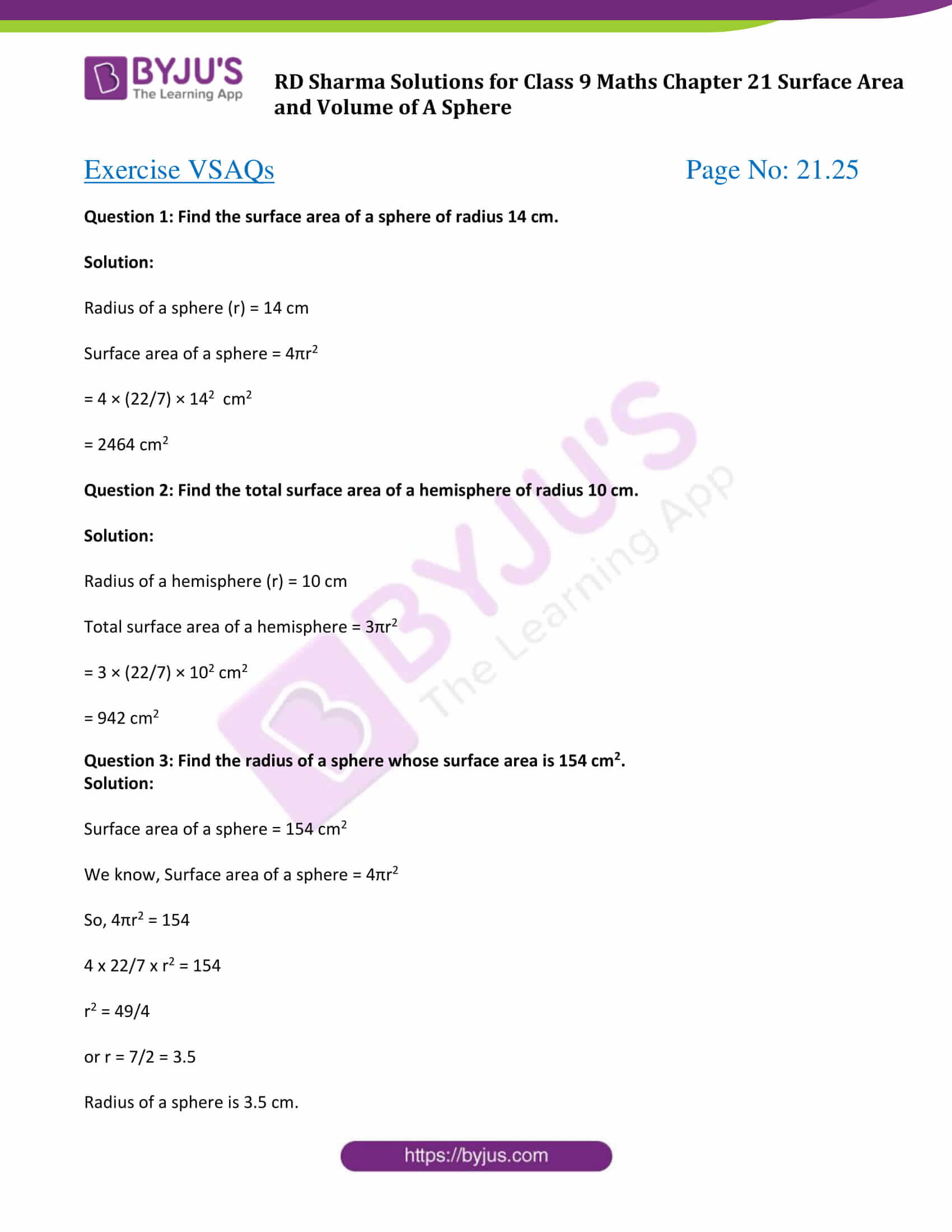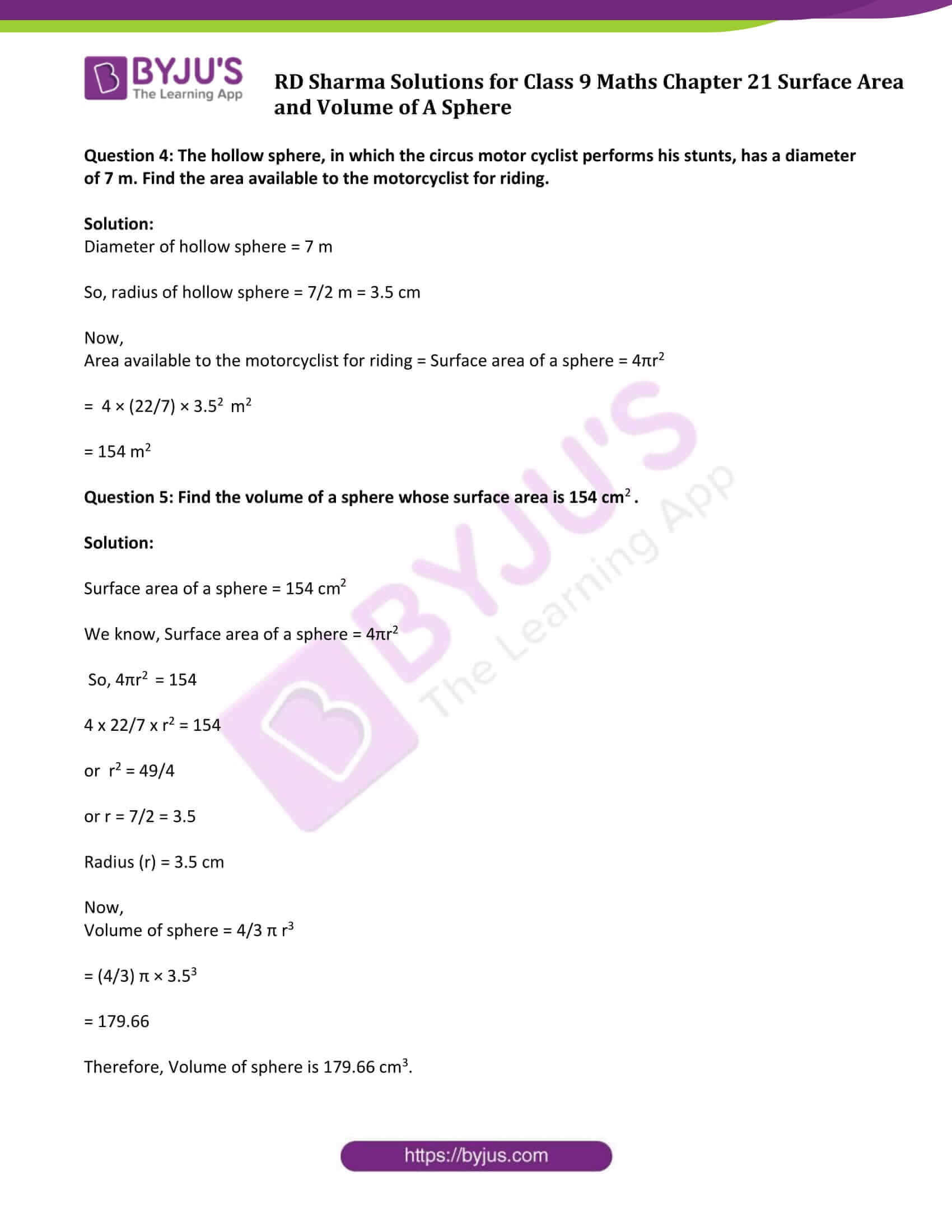# RD Sharma Solutions for Class 9 Maths Chapter 21 Surface Area and Volume of A Sphere Exercise VSAQs

Class 9 Chapter 21 – Surface Area and Volume of A Sphere Exercise VSAQs solutions are provided here. This topic comes under analytical geometry and the formulas for the volume and the surface area of the sphere was first discovered by Archimedes. You will be seeing questions using these formulas and with regular practice yields fruitful results. Students can also download exercise VSAQs pdf for future reference by following the given link.

## Download PDF of RD Sharma Solutions for Class 9 Maths Chapter 21 Surface Area and Volume of A Sphere Exercise VSAQs### Access Answers to Maths RD Sharma Solutions for Class 9 Chapter 21 Surface Area and Volume of A Sphere Exercise VSAQs Page number 21.25

Question 1: Find the surface area of a sphere of radius 14 cm.

Solution:

Radius of a sphere (r) = 14 cm

Surface area of a sphere = 4πr2

= 4 × (22/7) × 142 cm2

= 2464 cm2

Question 2: Find the total surface area of a hemisphere of radius 10 cm.

Solution:

Radius of a hemisphere (r) = 10 cm

Total surface area of a hemisphere = 3πr2

= 3 × (22/7) × 102 cm2

= 942 cm2

Question 3: Find the radius of a sphere whose surface area is 154 cm2.

Solution:

Surface area of a sphere = 154 cm2

We know, Surface area of a sphere = 4πr2

So, 4πr2 = 154

4 x 22/7 x r2 = 154

r2 = 49/4

or r = 7/2 = 3.5

Radius of a sphere is 3.5 cm.

Question 4: The hollow sphere, in which the circus motor cyclist performs his stunts, has a diameter of 7 m. Find the area available to the motorcyclist for riding.

Solution:

Diameter of hollow sphere = 7 m

So, radius of hollow sphere = 7/2 m = 3.5 cm

Now,

Area available to the motorcyclist for riding = Surface area of a sphere = 4πr2

= 4 × (22/7) × 3.52 m2

= 154 m2

Question 5: Find the volume of a sphere whose surface area is 154 cm2.

Solution:

Surface area of a sphere = 154 cm2

We know, Surface area of a sphere = 4πr2

So, 4πr2 = 154

4 x 22/7 x r2 = 154

or r2 = 49/4

or r = 7/2 = 3.5

Now,

Volume of sphere = 4/3 π r3

= (4/3) π × 3.53

= 179.66

Therefore, Volume of sphere is 179.66 cm3.

## RD Sharma Solutions for Class 9 Maths Chapter 21 Surface Area and Volume of A Sphere Exercise VSAQs

RD Sharma Solutions Class 9 Maths Chapter 21 Surface Area and Volume of A Sphere Exercise VSAQs is based problems to finding the volume and surface area of sphere using formulae. Below are some of the important formulas:

• Surface area of a sphere = 4πr2
• Surface area of the hemisphere = 2πr2
• surface area of solid hemisphere = 3πr2
• Volume of a sphere = 4/3πr3 Cubic Units
• Volume of hemisphere = 2/3 πr3

Where, r = radius of a sphere